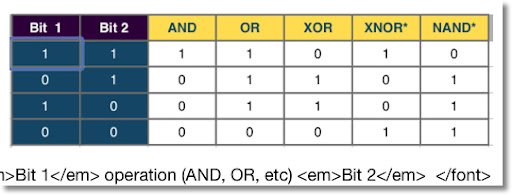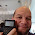## Thursday, May 3, 2012

### Logic Operators and the TI-36X Pro

Introduction

This blog entry is in response to a email regarding the logic operations I received from jcroot on the Texas Instruments TI-36X Pro Review: (link here)

Hello Eddie!

How are you today? I was just wondering if you could please give us an example of how to use the "Logic function" on this calculator, I am trying to follow the manual but I still can't understand in which situations this could be useful.

jcroot, I hope that this blog entry (at least in part) answers your question. I had to research a bit because I must confess that I do not use logic operators much on calculators.

Logic Operators

The most common logic operators are: and, or, not, 2's compliment and xor. The TI-36X has two additional operators xnor and nand. The latter two are not common on calculators.

I tend to think binary numbers as the prime example for these logic operators. It is my understanding that the operators compare the binary form (base 2 integers) of an integer and if necessary, convert the answer back into the required form (decimal, hexadecimal, or octal).

Binary Numbers

Binary numbers are numbers consisting of 0 and 1, with each digit being a bit. Often, the bit 0 is referred to "off" or "false", while the bit 1 is referred to "on" or "true". Each bit resembles a power of 2, with powers of 2 increasing from right to left:

...(2^n)...(2^5)(2^4)(2^3)(2^2)(2^1)(2^0)

For example, if the first (rightmost bit) is 1, then the value "1" is turned on. If the second bit is 1, then the value "2" is turned on, the third bit gives a value of "4", fourth bit gives a value of "8", and so on. Binary numbers are often written with a subscript of 2. On calculators, they are often designated with b or a "#b".

On the TI-36X Pro

Change to BINary Mode: Press [mode], arrow down five times, select BIN, hit [enter], then [2nd] [mode] (quit). You can designate DECimal (normal - base 10), HEXadecimal (base 16), and OCTal (base 8) modes in a similar way.

Designate a binary number in any mode: type your binary number, [2nd] [ 9 ] (base n), select TYPE, select 2: b. This procedure is not necessary if you are in Binary Mode.

[2nd] [ 9 ] (base n) gives you access to conversions (CONV), designations (TYPE), and operations (LOGIC).

One Argument Logic Operators

not: This operator basically switches a bit. In binary numbers, if the bit is 0, then not 0 = 1. Similarly, if the bit is 1, then not 1 = 0.

2's Complement: This complement is a way to manipulate binary numbers such that the arithmetic, specifically addition and subtraction of binary numbers, is accurate. More information is found by clicking on this sentence.

Two Argument Logic Operators

The following logic operations answer different types of questions:

and: Are both bit 1 true and bit 2 true?

or (inclusive or): Is either bit 1 or bit 2 true? Bit 1 and bit 2 can both be true.

xor (exclusive or): Is either bit 1 true or bit 2 true - without both bits being true?

xnor (not exclusive or): The opposite of xor. Are both bits true or both bits false?

nand (not and): The opposite of and. Is at least one of the bits false?

The following table summaries and, or, xor, xnor, and nand.How the TI-36X Works With Binary Numbers

All binary numbers work with 10 bit integers. Attempting to work integers greater than 10 bits causes a SYNTAX Error. This error is not mentioned in the TI-36X Manual.

The binary number of a TI-36X looks like this:

##########

where # is a 0 or 1. If you enter less than 10 bits, the calculator "fills" the leftmost bits with 0. Note that I designated the leftmost bit (10th bit) red and bold. This is known as the sign bit. If this bit is 0, then the integer is positive.

(sign) (2^8) (2^7) (2^6) (2^5) (2^4) (2^3) (2^2) (2^1) (2^0)
0 [ 1 = "on", 0 = "off"]

However, if the leftmost bit (10th bit) is 1, then the integer is negative. I am not 100% sure how the rest of the bits are designated.

Note in all examples, I designate binary numbers with the "b", like the calculator does.

10001b = 0000010001b = 17

1111101111b = -17

For the TI-36X Pro, the boundary for binary integers in the interval [-511, 511].
(2^9 - 1 = 511) Remember, the 10th bit is the sign bit on this calculator.

When using logic operators, the TI-36X compares each bit, including the 10th bit.

By the way, binary integers can be stored and recalled in variables.

Examples:

Let x = 0000011101b (5th, 4th, 3rd, and 1st bits are on, x = 29)
and y = 0000010100b (5th and 3rd bits are on, y = 20)

(decimal equivalents in parenthesis)
x and y = 0000010100b (29 and 20 = 20)
x or y = 0000011101b (29 or 20 = 29)
x xor y = 0000001001b (29 xor 20 = 9)
x xnor y = 1111110110b (29 xnor 20 = -10)
x nand y = 1111101011b (29 nand 20 = -21)

So, when I think of logic operations, I think of comparing binary numbers (or binary equivalent of integers) bit by bit, given a designated amount of bits.

Hope this helps,

Eddie.

This blog is property of Edward Shore. © 2012

1.Well done Eddie!

Many Thanks!

2.http://www.math-worksheets.co.uk/141-tmd-what-are-binary-numbers-part-1/

3.4.Thank you! This helped me understand logic in Intel processors. Didn't realize that the signed bit is digit 10, and not 8 as it is with a byte. Now I can calculate signed binary numbers properly.

5.what about after the decimal. for example 1011010101.10 I can do this by hand but us there a way to do this on the TI-36 Pro? I have looked everywhere.

6.Today I want to share my personal experience that helps my business. It is a great surprise for all traders. First time, I applied Pro Robot but I got bad output, loss and many time fake signals. Then I got free binary video tutorials of Trend Trader from my friend. The Trend Trader is new version, so easy, always provides me right time signal to buy or sell and auto earning. The Pro Robot gives me 15%”25% profit before Trend Trader gives me 55%”80% profit till now. I took my product price only 1 day by using The Trend Trader after that it brings only profit to me. I recommend you that Trend Trader has a customer support also. You can check by visiting their official website Trend Trader Official Website

7.Today I want to share my personal experience that helps my business. It is a great surprise for all traders. First time, I applied Pro Robot but I got bad output, loss and many time fake signals. Then I got free binary video tutorials of Trend Trader from my friend. The Trend Trader is new version, so easy, always provides me right time signal to buy or sell and auto earning. The Pro Robot gives me 15%”25% profit before Trend Trader gives me 55%”80% profit till now. I took my product price only 1 day by using The Trend Trader after that it brings only profit to me. I recommend you that Trend Trader has a customer support also. You can check by visiting their official website Trend Trader Official Website

### Retro Review and Comparison: TI-82 Advanced

Retro Review and Comparison: TI-82 Advanced I can officially say that I have a French calculator.   Quick Facts: Models:  TI-82 Advanced Co...